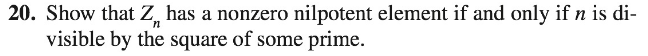# 20. Show that Z. has a nonzero nilpotent element if and only if n is di-visible by the square of some prime.

Question
1 views

Can someone please help me understand the following problem. I need to know how to start the problem. i need to know the theorems ,identities,  used please thank you.help_outlineImage Transcriptionclose20. Show that Z. has a nonzero nilpotent element if and only if n is di- visible by the square of some prime. fullscreen
check_circle

Step 1

Given: n is divisible by the square of prime.

To prove: Zn has a non zero nilpotent element.

Step 2

Assume the prime number, p

So,

n=p2t

For the element a=pt

(pt)2=p2t2

=nt

&...

### Want to see the full answer?

See Solution

#### Want to see this answer and more?

Solutions are written by subject experts who are available 24/7. Questions are typically answered within 1 hour.*

See Solution
*Response times may vary by subject and question.
Tagged in

### Math